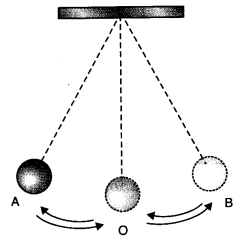Check the below Online Education NCERT MCQ Questions for Class 7 Science Chapter 13 Motion and Time with Answers Pdf free download. MCQ Questions for Class 7 Science with Answers were prepared based on the latest exam pattern. We have Provided Motion and Time Class 7 Science MCQs Questions with Answers to help students understand the concept very well. https://ncertmcq.com/mcq-questions-for-class-7-science-with-answers/

You can refer to NCERT Solutions for Class 7 Science Chapter 13 Motion and Time to revise the concepts in the syllabus effectively and improve your chances of securing high marks in your board exams.

## Online Education for Motion and Time Class 7 MCQs Questions with Answers

Motion And Time Class 7 MCQ Question 1.
A bus travels 54 km in 90 minutes. The speed of the bus is
(a) 0.6 m/s
(b) 10 m/s
(c) 5.4 m/s
(d) 3.6 m/s

Class 7 Science Chapter 13 MCQ Question 2.
If we denote speed by S, distance by D and time by T, the relationship between these quantities is
(a) S = D T
(b) T = $$\frac{S}{D}$$
(c) S = $$\frac{1}{T}$$ × D
(d) S = $$\frac{T}{D}$$

Answer: (c) S = $$\frac{1}{T}$$ × D

Time And Motion Class 7 MCQ Question 3.
Observe the figure given below:The time period of a simple pendulum is the time taken by it to travel from
(a) A to B and back to A
(b) O to A, A to B and B to A
(c) B to A, A to B and B to O
(d) A to B

Answer: (a) A to B and back to A

Class 7 Motion And Time MCQ Question 4.
Nearly all the clocks make use of
(a) straight line motion
(b) periodic motion
(c) random motion
(d) circular motion

MCQ On Motion And Time Class 7 Question 5.
A simple pendulum takes 42 sec. to complete 20 oscillations. What is its time period?
(a) 2.1 s
(b) 4.2 s
(c) 21 s
(d) 8.40 s

Class 7 Science Ch 13 MCQ Question 6.
Time period of a simple pendulum depends upon its
(a) weight of bob
(b) length
(c) both (a) and (b)
(d) None of these

MCQ Questions For Class 7 Science Chapter 13 Question 7.
Which of the following cannot be used for measurement of time?
(a) A leaking tap
(b) Simple pendulum
(c) Shadow of an object during the day

Motion And Time MCQ Class 7 Question 8.
On which axis is dependent variable represented?
(a) x-axis
(b) y-axis
(c) On any axis
(d) Depends on the data

Class 7 Science Motion And Time MCQ Question 9.
The correct symbol to represent the speed of an object is:
(a) 5 m/s
(b) 5 mp
(c) 5 m/s-1
(d) 5 s/m

Motion And Time Class 7 MCQ With Answers Question 10.
Boojho walks to his school which is at a distance of 3 km from his home in 30 minutes. On reaching he finds that the school is closed and comes back by a bicycle with his friend and reaches home in 20 minutes. His average speed in km/h is
(a) 8.3
(b) 7.2
(c) 5
(d) 3.6

Match the following:

 Column A Column B (i) Years (a) Rotation period of earth on its axis (ii) Hour (b) Time to complete 100 m race (iii) Minute (c) Gestation period in humans (iv) Days (d) Age of a person (v) Second (e) In scientific research (vi) Month (f) Age of stars (vii) Microseconds (g) Time to reach your school (h) Train reaches from one city to other

 Column A Column B (i) Years (d) Age of a person (ii) Hour (h) Train reaches from one city to other (iii) Minute (g) Time to reach your school (iv) Days (a) Rotation period of earth on its axis (v) Second (b) Time to complete 100 m race (vi) Month (c) Gestation period in humans (vii) Microseconds (e) In scientific research

Fill in the blanks:

1. The distance covered by an object in a ………………… is called its speed.

2. Time between one sunrise and the next sunrise was called a …………………

3. In a simple pendulum, the metallic ball suspended by thread is called its …………………

4. Time period of a pendulum depends on its …………………

5. The symbols of all units are written in …………………

6. A ………………… is one billionth of a second.

7. Motion of objects can be presented in pictorial form by their ………………… graph.

8. The distance-time graph for the motion of an object moving with a constant speed is a …………………

Choose the true and false statements from the following:

1. Faster vehicle has a higher speed.

2. All the clocks make use of some periodic motion.

3. The basic unit of speed is km/h.

4. The symbols of all units are written in singular.

5. The basic unit of time is second.

6. Age of a person is expressed in days.

7. The distance-time graph of standing vehicle is a straight line parallel to x-axis.